Next: Transient Oscillator Response Up: Damped and Driven Harmonic Previous: Driven Damped Harmonic Oscillation

# Driven LCR Circuits

Consider an LCR circuit consisting of an inductor,, a capacitor,, and a resistor,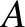, connected in series with an emf of voltage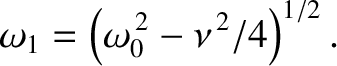. (See Figure 11.) Let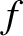be the instantaneous current flowing around the circuit. According to Kirchhoff's second circuital law, the sum of the potential drops across the various components of a closed circuit loop is equal to zero. Thus, because the potential drop across an emf is minus the associated voltage (Fitzpatrick 2008), we obtain [cf., Equation (90)]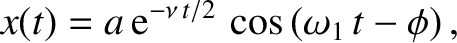(121)

where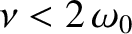. Suppose that the emf is such that its voltage oscillates sinusoidally at the angular frequency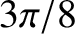, with the peak value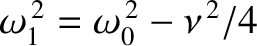, so that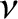(122)

Dividing Equation (121) by, and differentiating with respect to time, we obtain [cf., Equation (91)]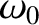(123)

where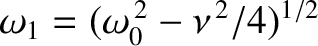and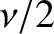. Comparison with Equation (101) reveals that this is a driven damped harmonic oscillator equation. It follows, by analogy with the analysis contained in the previous section, that the current driven in the circuit by the oscillating emf is of the form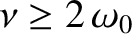(124)

where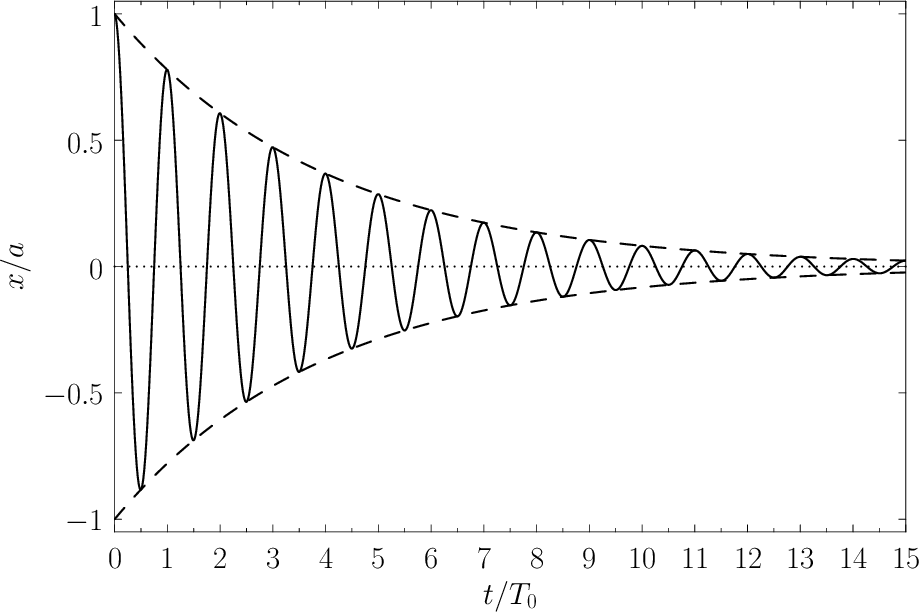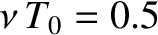(125)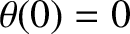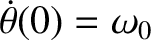(126)

In the immediate vicinity of the resonance (i.e.,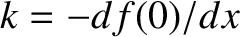), these expression simplify to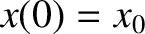(127)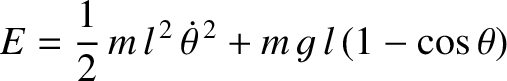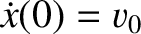(128)

The circuit's mean power absorption from the emf is written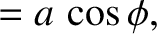(129)

so that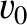(130)

close to the resonance. It follows that the peak power absorption,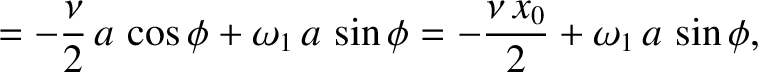, takes place when the emf oscillates at the resonant frequency,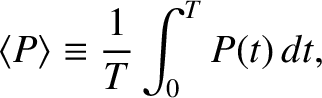. Moreover, the power absorption falls to half of this peak value at the edges of the resonant peak: that is,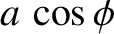.

LCR circuits can be used as analog radio tuners. In this application, the emf represents the analog signal picked-up by a radio antenna. According to the previous analysis, the circuit only has a strong response (i.e., it only absorbs significant energy) when the signal oscillates in the angular frequency range, which corresponds to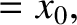. Thus, if the values of,, andare properly chosen then the circuit can be made to strongly absorb the signal from a particular radio station, which has a given carrier frequency and bandwidth (see Section 9.3), while essentially ignoring the signals from other stations with different carrier frequencies. In practice, the values ofandare fixed, while the value ofis varied (by turning a knob that adjusts the degree of overlap between two sets of parallel semicircular conducting plates) until the signal from the desired radio station is found.Next: Transient Oscillator Response Up: Damped and Driven Harmonic Previous: Driven Damped Harmonic Oscillation
Richard Fitzpatrick 2013-04-08